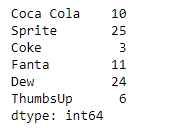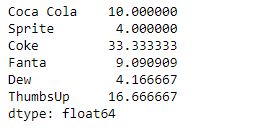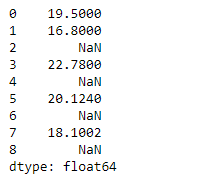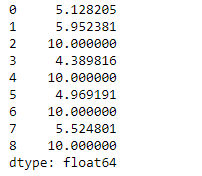# Python | Pandas Series.rdiv()

• Last Updated : 11 Feb, 2019

Pandas series is a One-dimensional ndarray with axis labels. The labels need not be unique but must be a hashable type. The object supports both integer- and label-based indexing and provides a host of methods for performing operations involving the index.

Pandas` Series.rdiv()` function perform the floating division of series and other, element-wise. The operation is equivalent to other / series, but with support to substitute a fill_value for missing data in one of the inputs.

Syntax: Series.rdiv(other, level=None, fill_value=None, axis=0)

Parameter :
other : Series or scalar value
fill_value : Fill existing missing (NaN) value
level : Broadcast across a level,

Returns : result : Series

Example #1: Use `Series.rdiv()` function to perform the floating division of a scalar with the given series object.

 `# importing pandas as pd``import` `pandas as pd`` ` `# Creating the Series``sr ``=` `pd.Series([``10``, ``25``, ``3``, ``11``, ``24``, ``6``])`` ` `# Create the Index``index_ ``=` `[``'Coca Cola'``, ``'Sprite'``, ``'Coke'``, ``'Fanta'``, ``'Dew'``, ``'ThumbsUp'``]`` ` `# set the index``sr.index ``=` `index_`` ` `# Print the series``print``(sr)`

Output :Now we will use `Series.rdiv()` function to perform the floating division of scalar with the series.

 `# divide the given value with series``result ``=` `sr.rdiv(other ``=` `100``)`` ` `# Print the result``print``(result)`

Output :As we can see in the output, the `Series.rdiv()` function has returned the result of floating division of the given scalar with the series object.

Example #2: Use `Series.rdiv()` function to perform the floating division of a scalar with the given series object. The given series object contains some missing values.

 `# importing pandas as pd``import` `pandas as pd`` ` `# Creating the Series``sr ``=` `pd.Series([``19.5``, ``16.8``, ``None``, ``22.78``, ``None``, ``20.124``, ``None``, ``18.1002``, ``None``])`` ` `# Print the series``print``(sr)`

Output :Now we will use `Series.rdiv()` function to perform the floating division of scalar with the series. We will fill 10 at the place of all the missing values in the series.

 `# divide the given value with series``result ``=` `sr.rdiv(other ``=` `100``, fill_value ``=` `10``)`` ` `# Print the result``print``(result)`

Output :As we can see in the output, the `Series.rdiv()` function has returned the result of floating division of the given scalar with the series object.

My Personal Notes arrow_drop_up